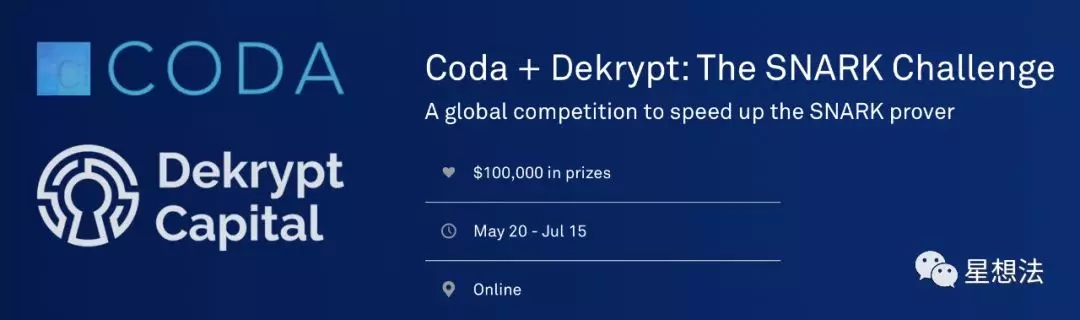### 零知识证明 - Coda SNARK挑战（Stage1）

「 最近事情有点多，没怎么写文章。最近好多朋友都在关心我，有没有参加Coda举办的零知识证明的挑战？必须的。不光为了奖金，整个挑战的内容本身也是好的学习材料。不光是我，整个团队都在梳理理论，GPU实现优化，小伙伴们都太给力了:)https://codaprotocol.github.io/snark-challenge/tutorial.html

## 01 第一题 - 域计算（大数模乘）

https://codaprotocol.github.io/snark-challenge/MNT4753.html

https://codaprotocol.github.io/snark-challenge/MNT6753.html

SNARK证明所需要的基本操作是乘法和加法。大数计算的位数比一般的计算使用的32位和64位要大的多。MNT4753和MNT6753的数为753位，也就是需要最少96个字节表示。753位，可以采用12个64位整数或者24个32位整数表示。其中的64位整数或者32位整数称为“limb”。

$\Bbb F q$的域由$0,1,...,q-1$组成。在这个域中可以进行加减乘除。以加法为例，a,b为$\Bbb F q$的两个数，则定义$a*b\ mod\ q$为a乘以b的结果。

## 04 第四题 - 椭圆曲线群运算

var curve_add = (p, q) => {
var s = (p.y - q.y) / (p.x - q.x);
var x = s*s - p.x - q.x;
return {
x: x,
y: s*(p.x - x) - p.y
};
};

http://www.hyperelliptic.org/EFD/g1p/auto-shortw-projective.html

 x=X/Z
y=Y/Z

 Y1Z2 = Y1*Z2
X1Z2 = X1*Z2
Z1Z2 = Z1*Z2
u = Y2*Z1-Y1Z2
uu = u2
v = X2*Z1-X1Z2
vv = v2
vvv = v*vv
R = vv*X1Z2
A = uu*Z1Z2-vvv-2*R
X3 = v*A
Y3 = u*(R-A)-vvv*Y1Z2
Z3 = vvv*Z1Z2

#### 总结：

Coda SNARK挑战的Stage1阶段介绍了SNARK算法的基础，大数算术（大数模乘）以及椭圆曲线群运算。SNARK挑战的本身也是很好的入门学习SNARK算法的好教材。

• 发表于 2019-06-28 10:17
• 阅读 ( 578 )
• 学分 ( 0 )
• 分类：零知识证明